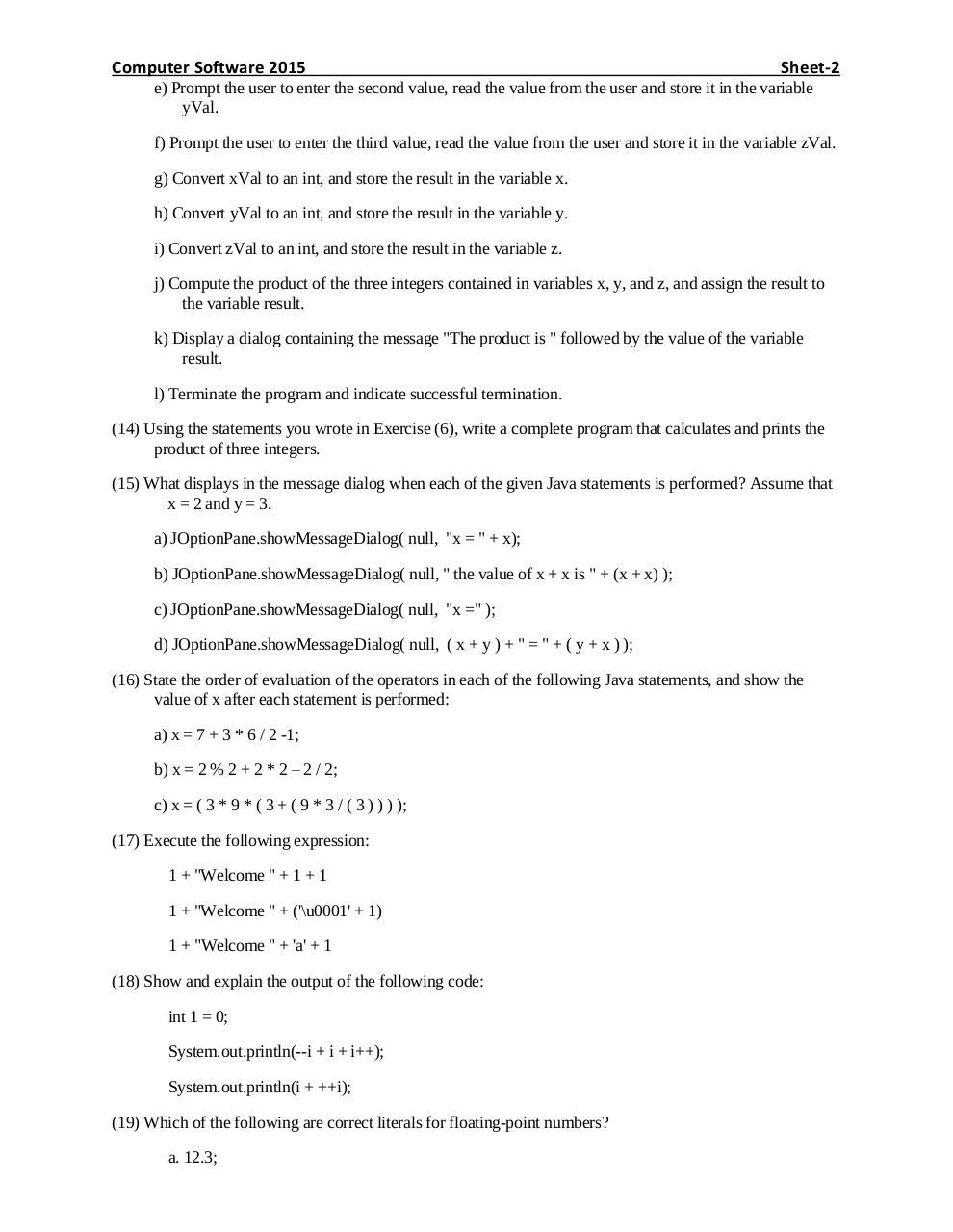Sheet 2 2015.pdfPage 1 2 3 4 5 6 7

Text preview

Computer Software 2015

Sheet-2

e) Prompt the user to enter the second value, read the value from the user and store it in the variable
yVal.
f) Prompt the user to enter the third value, read the value from the user and store it in the variable zVal.
g) Convert xVal to an int, and store the result in the variable x.
h) Convert yVal to an int, and store the result in the variable y.
i) Convert zVal to an int, and store the result in the variable z.
j) Compute the product of the three integers contained in variables x, y, and z, and assign the result to
the variable result.
k) Display a dialog containing the message &quot;The product is &quot; followed by the value of the variable
result.
l) Terminate the program and indicate successful termination.
(14) Using the statements you wrote in Exercise (6), write a complete program that calculates and prints the
product of three integers.
(15) What displays in the message dialog when each of the given Java statements is performed? Assume that
x = 2 and y = 3.
a) JOptionPane.showMessageDialog( null, &quot;x = &quot; + x);
b) JOptionPane.showMessageDialog( null, &quot; the value of x + x is &quot; + (x + x) );
c) JOptionPane.showMessageDialog( null, &quot;x =&quot; );
d) JOptionPane.showMessageDialog( null, ( x + y ) + &quot; = &quot; + ( y + x ) );
(16) State the order of evaluation of the operators in each of the following Java statements, and show the
value of x after each statement is performed:
a) x = 7 + 3 * 6 / 2 -1;
b) x = 2 % 2 + 2 * 2 – 2 / 2;
c) x = ( 3 * 9 * ( 3 + ( 9 * 3 / ( 3 ) ) ) );
(17) Execute the following expression:
1 + &quot;Welcome &quot; + 1 + 1
1 + &quot;Welcome &quot; + ('\u0001' + 1)
1 + &quot;Welcome &quot; + 'a' + 1
(18) Show and explain the output of the following code:
int 1 = 0;
System.out.println(--i + i + i++);
System.out.println(i + ++i);
(19) Which of the following are correct literals for floating-point numbers?
a. 12.3;